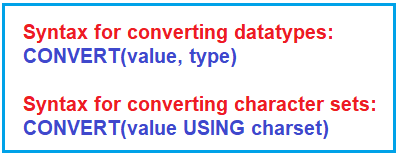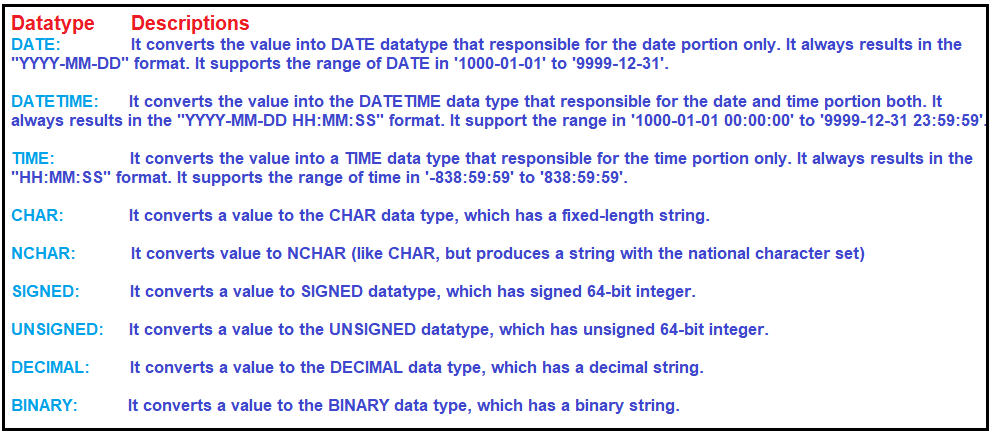# MySQL CONVERT Function

## MySQL CONVERT Function with Examples

In this article, I am going to discuss MySQL CONVERT Function with Examples. Please read our previous article where we discussed MySQL CAST Function with Examples.

##### MySQL CONVERT Function

Similar to the CAST function, the MySQL CONVERT function is used to convert a value or expression from one data type to another data type. It is also used to converts a value or expression from one character set to another character set.

###### Syntax:

Following is the syntax to use the CONVERT Function. The MySQL CONVERT function accepts two parameters as shown in the below image.Parameters

1. value: Required: This is the value that needs to be converted.
2. type: Required: It is used to specify the desired data type to which the value needs to be converted.
3. Charset: Required: It is used to specify the desired character set to be converted in.

Return Value: The CONVERT function returns a value in the desired datatype or character set after conversion.

following are the datatypes to which the MySQL CONVERT function works perfectly:##### Examples:

Let us understand the need and use of the Convert function with some examples.

Example: Converting a value to DATE datatype using the CONVERT function.
SELECT CONVERT(“2020-10-25”, DATE) AS ConvertedDate;
Output: 2020-10-25

Example: Converting a value to DATETIME datatype using the CONVERT function.
SELECT CONVERT(“2020-10-25”, DATETIME) AS ConvertedDateTime;
Output: 2020-10-25 00:00:00

Example: Converting a value to TIME datatype using the CONVERT function.
SELECT CONVERT(“2020-10-25 08:14:57”, TIME) AS ConvertedTime;
Output: 08:14:57

Example: Converting a value to CHAR datatype using the CONVERT function.
SELECT CONVERT(‘123’, CHAR) AS ConvertedChar;
Output: 123

Example: Converting a value to NCHAR datatype using the CONVERT function.
SELECT CONVERT(‘123’, NCHAR) AS ConvertedNChar;
Output: 123

Example: Converting a value to SIGNED datatype using the CONVERT function.
SELECT CONVERT(20 – 44, SIGNED) AS ConvertedSigned;
Output: -24

Example: Converting a value to UNSIGNED datatype using the CONVERT function.
SELECT CONVERT(20 – 44, UNSIGNED) AS ConvertedUnsigned;
Output: 18446744073709551592

Example: Converting a value to DECIMAL datatype using the CONVERT function.
SELECT CONVERT(‘4.4’, DECIMAL(6,2)) AS ConvertedDecimal;
Output: 4.40

Example: Converting a string value to utf8 character set using the CONVERT function
The second syntax for the MySQL CONVERT function converts a value from one character set to another character set. In the following example, the CONVERT function takes the value ‘MySQLTutorials’ and converts it from the current character set to the utf8 character set.
SELECT CONVERT(‘MySQLTutorials’ USING utf8) AS ConvertedCharacterSet;
Output: MySQLTutorials

Example: Converting a string value to latin1 character set using CONVERT function.
In the following example, the CONVERT function takes the value ‘MySQLTutorials’ and converts it from the current character set to the latin1 character set.
SELECT CONVERT(‘MySQLTutorials’ USING latin1) AS ConvertedCharacterSet;
Output: MySQLTutorials

##### Difference between CAST AND CONVERT Function

The main difference between MySQL CAST and CONVERT functions are given below.

###### CAST Function:
1. We use the MySQL CAST function to convert one data type into the other data type.
2. It cannot be used to convert a character set.
3. It is the part of ANSI-SQL standard
4. CAST function uses the “AS” keyword for separating the parameter.
###### CONVERT Function:
1. We use the MySQL CONVERT function to convert one data type into the other data type.
2. It can be used to convert a character set.
3. It is not part of the ANSI-SQL standard
4. CONVERT function uses the “comma(,)” or “USING” keyword for separating the parameter.